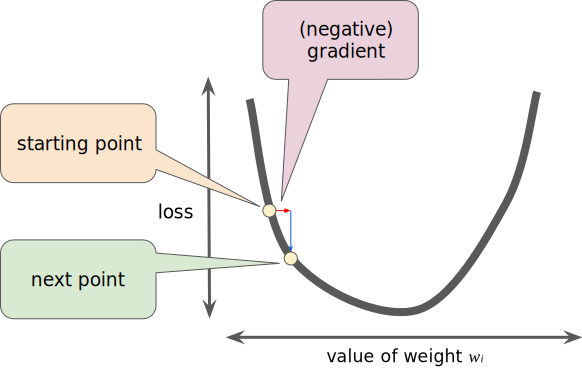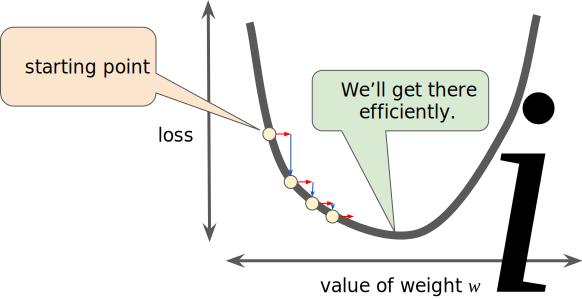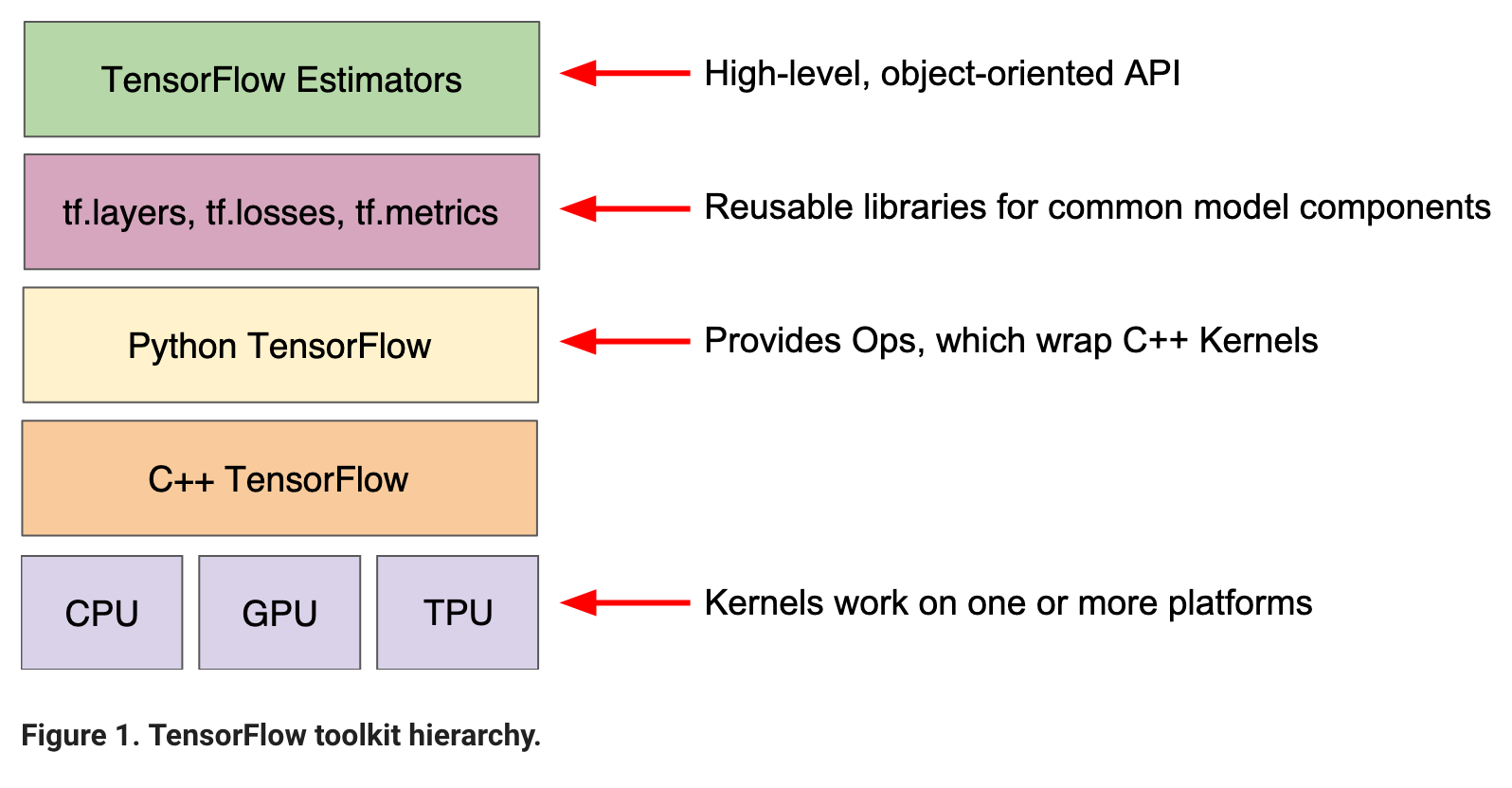# Deep Learning concepts

### Terminology¶¶

When in DL, terms such as intercept and slope of linear regression are called as bias and weight vectors. The process by which the ML algorithm (linear regression in this case) reduces errors (also called loss) is called empirical risk minimization.

$L_{2} loss$ is the loss function that we call squared loss or MSE. L2 is popular in ML.

### Reducing loss¶¶

Hyperparameters are the config settings used to tune how the model is trained. The derivative of (y-y')^2 (derivative of sq. error) with respect to the weights and biases shows how loss would change. The model reapeatedly takes small steps in the direction that minimizes loss. This process is called Gradient Descent.

The way the ML engine reduces loss is similar to how a kid plays the game of “Hot or Cold”. The engine starts off by setting random values for the weights and bias, then calculates the loss. It tweaks the weights and observes how the loss changes. Here the loss function (L2 or Sq. Error, MSE, RMSE are examples of loss functions). When the overall loss changes very less or does not reduce at all, we say the model has converged.

To calculate the gradient descent, the ML algorithm has to calculate the gradient of the loss function. This is via partial differential equations, differntiating the loss function with respect to one variable (parameter) at a time. The negative of the gradient (slope) tells it in which direction to change the variable to minimize loss.

In ML, loss or loss function is the function used to quantify errors. $L_{2}$ loss is the squared error in prediction. When an ML algorithm tries to minimize this loss, it iterates over many values for the weights. Instead of doing a brute force series of attempts, you can represent the loss as a curve and try to find its minima.

The process of traversing this curve to find the point of minimal loss is called gradient descent.#### Learning rate¶¶

Learning rate controls how large the steps should be as the model moves toward lower gradient. A large learning rate will make the model overshoot the local minima of loss and would never converge. A small learning rate would require way too many iterations to converge. An optimal learning rate is required.

Gradient vector has both the direction and magnitude. GD algorithms multiply the gradient vector with a scalar known as learning rate to determine the next point.

A large learning rate (step size) will cause the algorithm to zig zag and easily over shoot the minima of loss. A too small LR will slow down and GD process and never allow it to converge. An optimal LR will try to arrive at the minima with fewest possible iterations.Stochastic gradient descent: To calculate the gradient, you need to compute the gradient for all input datasets. However, empirically just doing this on a small subset of training data also yields comparable results. Thus the name stochastic gradient descent. Per this tutorial, stochastic is when you compute on just 1 training sample and mini-batch gradient descent is when you compute on a mini batch of training samples.

Through central limit theorem, we know performing GD on many tiny datasets and averaging them would give us a fair estimate of true GD. Thus, SGD takes this to the extreme and computes GD on just 1 dataset, but over multiple such singleton samples.

Mini batch SGD is a compromise, where GD is computed over batches of size between 10 - 1000. Mini batch SGD is smoother than SGD, but performs faster than running a GD.

### Tensorflow architecture¶¶While using TF, the estimator API (tf.estimator) provides a high-level OOP API. The middle layers such as tf.layers / tf.losses / tf.metrics provide a library of common model components. Then the TensorFlow module gives you lower level API.

The foundation of computation in Tensorflow is a Graph object which is a network of nodes. Each node contains input, operation and output. Once you built a graph, you can save it as a GraphDef object. This object can be serialized to disk (using protocol buffer format) as a TF model file. These files are binary, but you can save them out as text files as well.

In [ ]: